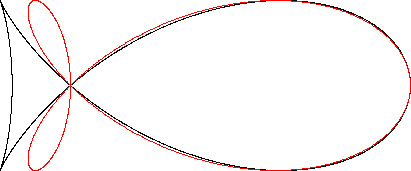Trefoil
TREFOIL

Balmoral Software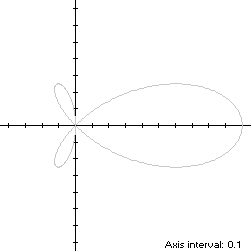The trefoil S is defined by the x-symmetric polar equation
r(t) = cos(t)cos(2t)
for t ∈ T = [0,π). The curve always moves counterclockwise, visiting the origin three times, hence its name. At t = 0, the trefoil starts at its maximum abscissa point (1,0) and moves through Quadrant I along its main lobe to its maximum ordinate point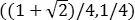at t = π/8, then on to the origin at t = π/4. It continues along a small lobe in Quadrant III, returning to the origin at t = π/2. Next, it travels along its other small lobe in Quadrant II to the same ordinate maximum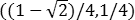at t = 5π/8 and one of its minimum abscissa points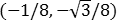at t = 2π/3, before going back to the origin at t = 3π/4. It then returns along the main lobe through Quadrant IV to its starting point at t = π. S is non-convex by the multiple local extrema test, and the width x height of its bounding rectangle is 9/8 x 1/2.

### Metrics

The area of the trefoil is π/8. We have
r'(t) = -[sin(t) + 3sin(3t)]/2,
so by (L2), the perimeter of S is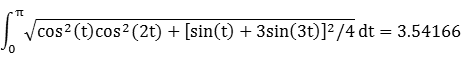Its centroid abscissa is 1/2.

### Convex Hull

The convex hull is created by connecting the extreme ordinate and abscissa points with two horizontal line segments of length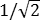and one vertical line segment of length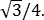By (L2), the perimeter of the convex hull consists of these line segments plus the arc of a thin wedge in each small lobe and the convex portion of the main lobe: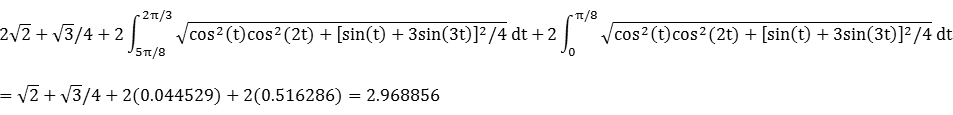The perimeter of the convex hull is about 16% shorter than that of the trefoil.

In the upper half-plane, the line segments of the convex hull create two triangles, shown in blue in the left diagram at the bottom of this page. At left is a right triangle of area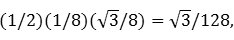and whose centroid abscissa -1/12 is the average of its vertex abscissas. At the top is a scalene triangle with area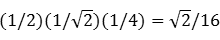and centroid abscissa 1/6. By (A2), the thin wedge of the small lobe has areaBy (C1), its centroid abscissa is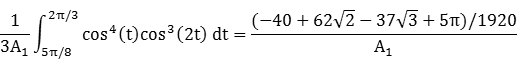Similarly, the area of the convex portion of the main lobe in the upper half-plane is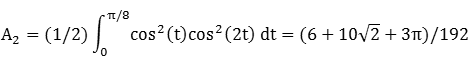and its associated centroid abscissa is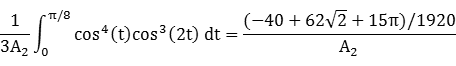The component metrics for half of the convex hull can be summarized as follows:
 Area Centroid abscissa Product Region Right triangle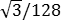-1/12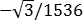Small lobe wedge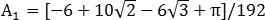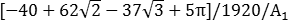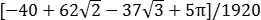Scalene triangle1/6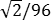Main lobe portion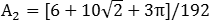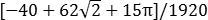Total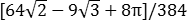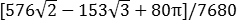The total area of the convex hull is 0.521114, about 1/3 larger than that of the trefoil. The centroid abscissa of the convex hull is the weighted average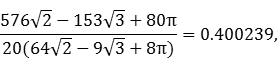### Circumcircle

We have
x(t) = r(t)cos(t) = cos2(t)cos(2t)

y(t) = r(t)sin(t) = sin(4t)/4

Using z = 1 in Lemma C,
[x2(t) + y2(t) - z2]/[x(t) - z]/2 = cos(2t)/2 + 1/[cos(2t) + 2]
is minimized atFor verification, we have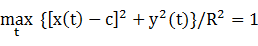### Circumellipse

Lemma E does not produce an ellipse that fully contains S, so we resort to a numerical search to determine the optimal circumellipse:
a = 0.698874

b = 0.312453

c = 0.312454

d = 0

It's interesting to note that the circumellipse's center abscissa c is the same as its half-height b in this optimal solution.

### Incircle (main lobe)

The maximum radius of a circle centered on the x-axis and inscribed in the trefoil's main lobe is its maximum ordinate, so a candidate for the incircle has radius R = 1/4 = 0.25 and center abscissaThe candidate circle must be contained within S, so we require that c - R and c + R both be within the abscissa range [0,1] of S, which is true. For verification, we have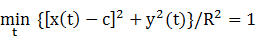### Inellipse (main lobe)

Using z = 1 in Lemma E,
d/dt [x(t) - z]y(t) = d/dt [cos2(t)cos(2t) - 1]sin(4t)/4
has a zero at t* = 0.538406 radians. The corresponding coordinates are
x* = 0.349472

y* = 0.208727

We then have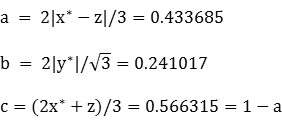For verification,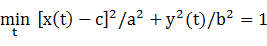### Tail

As in the case of the lobes of the bifolium, the two small lobes in the tail of the trefoil are symmetric to each other, but each is asymmetric with respect to its maximum-radius axis (spine). To see this, rotate the trefoil clockwise so that the spine of its upper small lobe is oriented along the x-axis. To determine the angle of rotation, we begin by finding the maximum radius point of the trefoil in Quadrant II (π/2 ≤ t ≤ 3π/4), which occurs at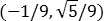when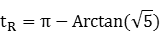Since the trefoil is defined by a polar function, tR is also the clockwise rotation angle. The parametric coordinate functions of the rotated curve are: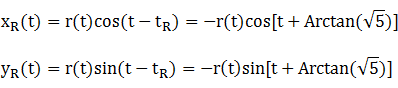The asymmetry can then be seen by comparing the upper half of the lobe (red) with a reflection of the lower half (blue):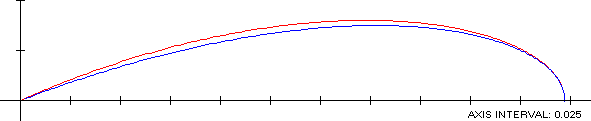### Incircle (tail)

Since each tail lobe is asymmetric, we resort to an unconstrained numerical search over the lobe parameter range π/4 ≤ t < π/2 to determine the optimal incircle for the lower tail lobe:
R = 0.038767

c = -0.070559

d = -0.160962

### Inellipse (tail)

The inellipse of the upper tail lobe can be found by first performing an unconstrained numerical search of the rotated lobe defined by the equations above over the parameter range π/2 ≤ t < 3π/4 to determine an optimal axis-aligned inellipse with an off-axis center:
a = 0.111756

b = 0.036490

c = 0.160167

d = -0.001576

To display the tilted ellipse in the upper tail lobe, the axis-aligned ellipse is rotated counterclockwise by tR = 114.094843° to produce the result in the left-hand diagram below. This approach presupposes that the major axis of the ellipse is along the spine of the lobe, which produces a slightly suboptimal inellipse.

### Summary Table

 Perimeter Area Centroid Figure Parameters Incircle (tail) R = 0.038767 0.243580 0.004721 (-0.070559,-0.160962) Inellipse (tail) * a = 0.111756b = 0.036490 0.496259 0.012811 (0.160167,-0.001576) Incircle (main lobe) R = 1/4 1.570796 0.196350 (0.603553,0) Inellipse (main lobe) a = 0.433685b = 0.241017 2.163075 0.328376 (0.566315,0) Trefoil Width: 9/8Height: 1/2 3.54166 0.392699 (0.5,0) Convex hull 2.968856 0.521114 (0.400239,0) Circumellipse a = 0.698874b = 0.312453 3.294240 0.686015 (0.312454,0) Circumcircle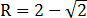3.680605 1.078024 (0.414214,0)

*: With respect to rotated curve S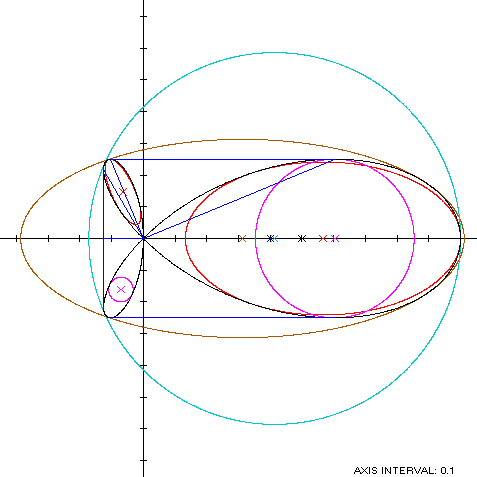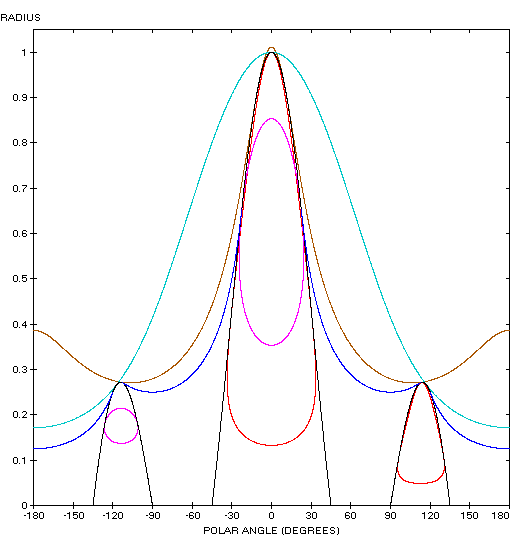The trefoil (red) is similarly shaped to the fish curve: